## Categories and Functors

"general abstract nonsense", "functors leave complexes complex"

category
A category C consists of a family Ob C of objects, and for each A,B∈Ob C a set MorC(A,B) of morphisms between A and B such that
 r ∀A∈Ob C ∃idA∈MorC(A,A) reflexive, identity t ∀A,B,C∈Ob C ∃∘:MorC(B,C)×MorC(A,B)→MorC(A,C) with transitive, composition n ∀A,B∈Ob C ∀g∈MorC(A,B) g∘idA = g neutral ∀A,B∈Ob C ∀f∈MorC(B,A) idA∘f = f a ∀A,B,C,D∈Ob C ∀f∈MorC(A,B)∀g∈MorC(B,C)∀h∈MorC(C,D) (h∘g)∘f = h∘(g∘f) associative
We usually assume that MorC(A,B) ∩ MorC(C,D) = ∅ unless A=C ∧ B=D.
Write: f:AB for f∈MorC(A,B)

Beware: Unlike MorC(A,B), Ob C does not need to be a set of objects, but only a class of objects. Nevertheless we formally write ∈,⊆ etc.

covariant
functor
A covariant functor F:CD between two categories C and D consists of a map F:Ob C→Ob D and for each A,B∈Ob C a map F:MorC(A,B)→MorD(F(A),F(B)) that satisfies the following conditions
 1 ∀A∈Ob C F(idA) = idF(A) unital m ∀f∈MorC(A,B)∀g∈MorC(B,C) F(g∘f) = F(g) ∘ F(f) morph

Beware: F:CD is not a map, since C,D are no sets (they are categories) and even Ob C,Ob D need not be sets.

contra-
variant
functor
A contravariant functor F:CD between two categories C and D consists of a map F:Ob C→Ob D and for each A,B∈Ob C a map F:MorC(A,B)→MorD(F(B),F(A)) that satisfies the following conditions
 1 ∀A∈Ob C F(idA) = idF(A) unital m° ∀f∈MorC(A,B)∀g∈MorC(B,C) F(g∘f) = F(f) ∘ F(g) contra-morph
A contravariant functor F:CD is a covariant functor F:C (or F:C°D).
natural
trans-
formation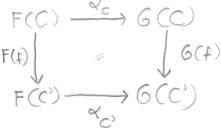A natural transformation (or morphism of functors) α:FG between the functors F,G:CD is a family (αC∈MorD(F(C),G(C)))C∈Ob C of morphisms such that
∀f∈MorC(C,C') G(f)∘αC = αC'F(f)
The functors CD form a category with natural transformations being the morphisms.
Remark: an isomorphism of functors is just a family of isomorphisms (αC∈IsoD(F(C),G(C)))C∈Ob C with the above property (⇒ especially F(C)≅G(C) ∧ G(f) = αC'F(f)∘αC-1).

The elements of the monoid MorC(A,A) are called endomorphisms of A. The elements of MorC(A,B) that have an inverse in MorC(B,A) are called isomorphisms. The elements of the group of isomorphisms in MorC(A,A) are called automorphisms.

 Let f∈MorC(A,A') be a morphism. monomorphism ∀B∈Ob C mB(f) injective f is left cancelable ⇔ f injective (if C=Ens ) epimorphism ∀B∈Ob C mB(f) injective f is right cancelable ⇔ f surjective (if C=Ens )
kernel ≤ equalizer
i:XA is a kernel of f:AB :⇔ f∘i = 0, and ∀j:X'A with f∘j=0 ∃!j':X'X such that the diagram commutes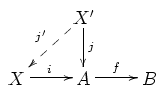.
Write: i = ker(f)
cokernel ≤ coequalizer
p:BY is a kernel of f:AB :⇔ p∘f = 0, and ∀j:BY' with j∘f=0 ∃!j':YY' such that the diagram commutes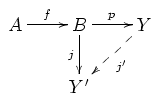.
Write: p = coker(f)
A category C is additive, if
 ∀A,B∈Ob C HomC(A,B) := MorC(A,B) is an Abelian(!) group d ∘ is distributive over +, i.e. HomC(·,B) and HomC(B,·) are functors C→Ab ∀A,B,C∈Ob C ∀f∈HomC(B,C) ∀g,h∈HomC(A,B) f∘(g+h) = (f∘g) + (f∘h) ∀A,B,C∈Ob C ∀f,g∈HomC(B,C) ∀h∈HomC(A,B) (f+g)∘h = (f∘h) + (g∘h) distributive
A functor F:CD between two additive categories C and D is additive, if
A,B∈Ob C F:HomC(A,B)→HomD(F(A),F(B)) homomorphism of groups
⇒ ∀GF G is additive
faithful
A functor F is faithful, if it is injective on maps.
full
A functor F is full, if it is surjective on maps.
Abelian
A category C is Abelian, if
 C is additive all coproducts, kernels and cokernels exist. (⇒ short exact sequences exist) Isomorphism Theorem. every monomorphism is the kernel of its cokernel (m = ker coker m), every epimorphism the cokernel of its kernel (f = coker ker f). normal
 C has a zero object all finitary limits and colimits exist every monomorphism is the kernel of its cokernel (m = ker coker m), every epimorphism the cokernel of its kernel (f = coker ker f). normal

### Important Examples

1. For every category C, there is the opposite (dual) category obtained by "turning the arrows around", per Ob := Ob C, and Mor(A,B) := MorC(B,A), whereas the identity morphisms are the same, and the composition is f∘g := g∘Cf.
Duality Principle: Any theorem holding for a category C also holds (in ) with all arrows reversed.

2. Categories together with functors form a category.

3. Additive categories form a category together with additive functors.

4. Functors of fixed categories together with their natural transformations form a category.

5. Let C be a category. For each X∈Ob C there are two functors

• covariant hom-functor  mX:=MorC(X,·) C → Ens Y ↦ Y∗:=MorC(X,Y) (f∈MorC(Y,Z)) ↦ (MorC(X,Y)→MorC(X,Z); g↦f∘g)
• contravariant hom-functor
 mX:=MorC(·,X) C → Ens Y ↦ Y∗:=MorC(Y,X) (f∈MorC(Y,Z)) ↦ (MorC(Z,X)→MorC(Y,X); g↦g∘f)

They result from currying ∘. The properties of being a functor coincides with the properties of morphisms (n) and (a), here. mX is the opposite of mX. If C is additive we write HomC(X,·):=hX:=mX, and HomC(·,X):=hX:=mX for these (now additive) functors in CAb. The properties of being additive coincides with (d).

6. Let C be a category, and S∈Ob C. Then C/S is the category of S-objects in C with
Ob C/S := {(X,f) ¦ X∈Ob C, f ∈MorC(X,S)}
MorC/S((X,f),(Y,g)) := {φ∈MorC(X,Y) ¦ f = g ∘ φ}

### Further Terminology

Yoneda-Lemma
Let F be the category of functors CEns
⇒ ∀A,B∈Ob C the following map is bijective
α MorC(A,B) →̃ MorF(mB,mA)
τB(idB) τ
φ
 αφ mB → mA (αφ)C MorC(B,C) → MorC(A,C) f ↦ f∘φ

If C is additive and F the category of (additive?) functors CAb, then α is an isomorphism of groups.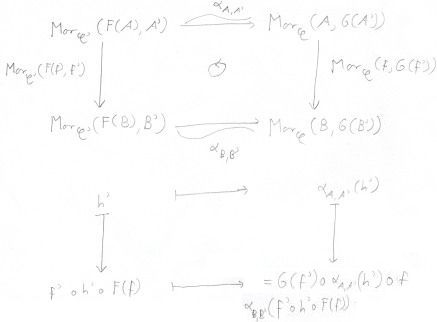F:CC' left-adjoint to G:C'C (and G right-adjoint to F) :⇔
mF := MorC'(F(·),·) ≅ MorC(·,G(·)) =: mG in C°×C'Ens
"what F does to the source (adding primes) is what G does to the domain (removing primes)"
equivalent
categories
F:CC' is an equivalence of categories :⇔
G:C'C with FG≅idC'GF≅idC
F left-adjoint to G and conversely
Ismomorphic categories are equivalent.

For the following definition, A and A' must be categories that have exact sequences (more precisely: Abelian categories) .

exact
F:AA' is exact :⇔ F:AA' is a (covariant) additive functor, and
 If 0 → A' →α A →β A'' → 0 is an exact sequence in A Then 0 → F(A') →F(α) F(A) →F(β) F(A'') → 0 is an exact sequence in A'
That the image under F of the sequence (or any complex) is a complex, is always true since F is a functor.
left exact
F:AA' is left exact :⇔ F:AA' is a (covariant) additive functor, and
 If 0 → A' →α A →β A'' (→ 0) is an exact sequence in A Then 0 → F(A') →F(α) F(A) →F(β) F(A'') is an exact sequence in A'.
right exact
F:AA' is right exact :⇔ F:AA' is a (covariant) additive functor, and
 If (0 →) A' →α A →β A'' → 0 is an exact sequence in A Then F(A') →F(α) F(A) →F(β) F(A'') → 0 is an exact sequence in A'.
F:AA' is right exact ⇔ F:A'° is left exact.
injective
I∈Ob A is injective :⇔ HomA(·,I) exact
⇔ ∀A'AAα':A'→I ∃α:A→I which is a continuation of α'.
A has sufficiently many injective objects :⇔ each object is a subobject of an injective object.
projective
P∈Ob A is projective :⇔ HomA(P,·) exact
⇔ ∀β:B→B'' surjective ∀γ:P→B'' ∃γ̃:P→B which is a lifting, i.e. β∘γ̃ = γ.
A has sufficiently many projective objects :⇔ each object is a quotient of a projective object.

## Universal Elements

Let C be a category.

terminal
object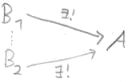A∈Ob C is a terminal object :⇔ ∀B∈Ob C ∃!φ∈MorC(B,A) ⇔ A is an initial object in .
terminal objects are uniquely isomorph, i.e. each two terminal objects A,B have a unique isomorphism A→B.
Terminal objects are the limits of the empty category.
initial
object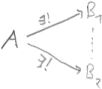A∈Ob C is an initial object :⇔ ∀B∈Ob C ∃!φ∈MorC(A,B)
initial objects are uniquely isomorph, i.e. each two initial objects A,B have a unique isomorphism A→B. Initial objects are the colimits of the empty category.
zero
object
A∈Ob C is a zero object :⇔ A is an initial and terminal object.
presentable
a covariant (resp. contravariant) functor F:CEns is presentable :⇔ ∃A∈Ob C FmA (resp. FmA)
A is called presenting object for F.
universal element
If F:CEns is a presentable covariant functor with a corresponding isomorphism of functors α:mAF, then uF := αA(idA) ∈ F(A) is called universal element of F .
⇒ ∀B∈Ob C ∀x∈F(B) ∃!f∈MorC(A,B)=mA(B) x = F(f)(uF)
• (The converse is also true)
•  if C and Ens (then Ab) are additive, then (mA,F are and) the αB are isomorphisms of groups.
• More generally, if F (on morphisms) is a homomorphism of a law + that ∘ is distributive over, then the αB are isomorphisms of +.
Let F:CEns be a presentable covariant functor.
• presenting objects for F are uniquely isomorph. Precisely: for all isomorphisms of functors α:mAF, α':mA'F ∃!φ:A→A' isomorphism with α∘αφ = α'. (Because of Yoneda-Lemma).
• an isomorphism of functors α:mAF is uniquely determined by its universal element, i.e. ∀u∈F(A) there is at most one α:mAF with αA(idA)=u.

## Product and Coproduct

Let C be a category, and I be a set.
product ≤ limit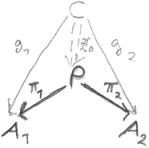P∈Ob C, with the projectors πi∈Mor(P,Ai) for i∈I, is a product of the objects (Ai)i∈I⊆Ob C :⇔
(∀C∈Ob C(gi∈MorC(C,Ai))i∈I
∃!g∈MorC(C,P) ∀i∈I πi∘g = gi )
Write: P = ∏i∈IAi
"product is universally attracting", and product is the presenting object of the contravariant functor CEns; C ↦ Mor(C,A1)×Mor(C,A2) with universal projector maps πi. Furthermore, it is just a terminal object in a suitable category. Products are the limits of a functor from a discrete category (i.e. which only has identity morphisms and thus reduces to a family of objects).
coproduct ≤ colimitS∈Ob C, with the inclusions ιi∈Mor(Ai,S) for i∈I, is a coproduct of the objects (Ai)i∈I⊆Ob C :⇔
(∀C∈Ob C(gi∈MorC(Ai,C))i∈I
∃!g∈MorC(S,C) ∀i∈I g∘ιi = gi )
Write: S = ∐i∈IAi (= ∑i∈IAi, sometimes ⊕i∈IAi)
"coproduct is universally repelling", and it is just an initial object in  a suitable category. A coproduct in C is just a product in .
fibred-product
X ×S Y is a fibred product of X ∈ Ob C and Y∈Ob C over S∈Ob C if it is a product of (X,f) and (Y,g) in the category C/S of S-objects. Also called pullback: "parallel translation". Pullbacks are the limits of a three-object category with f:X→S, g:Y→S as non-identity morphisms. They visualize as commutative squares with diagonal.
equalizer ≤ limit
the map e∈Mor(E,X), with E∈Ob C, is an equalizer of the maps f,g∈Mor(X,Y) :⇔
f∘e = g∘e
(∀E'∈Ob C ∀e'∈MorC(E',X) f∘e' = g∘e' ⇒
∃!η∈MorC(E',E) e' = e∘η )
e is a unique monomorphism (up to isomorphism). f.ex. The kernel of f is the equalizer of f and 0. Equalizers are the limits of the identity functor from a two-object category with two parallel morphisms in between.
coequalizer ≤ colimit
the map c∈Mor(Y,C), with C∈Ob C, is a coequalizer of the maps f,g∈Mor(X,Y) :⇔
c∘f = c∘g
(∀C'∈Ob C ∀c'∈MorC(Y,C') c'∘f = c'∘g ⇒
∃!γ∈MorC(C,C') c' = γ∘c )
c is a unique epimorphism (up to isomorphism). f.ex. The cokernel of f is the coequalizer of f and 0.

As terminal or initial objects, products and coproducts are uniquely determined up to a unique isomorphism. Even more so as they are special cases of limits and colimits.

## Limits and Colimits

Let C, J be categories.
limit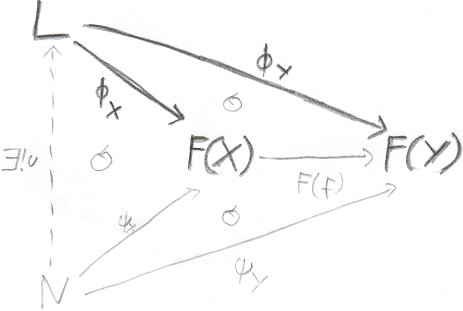L∈Ob C, together with φX∈MorC(L,F(X)) for each X∈Ob J, is a cone of the covariant functor F:JC  :⇔
∀f∈MorJ(X,Y) F(f)∘φXY
A limit of the covariant functor F:JC is a universal cone (L,(φX)X∈Ob J), i.e. the morphisms of any cone factor through L with the unique factorization u, i.e.
∀(N,(ψX)X∈Ob J) cone of F ∃!u∈MorC(N,L) ∀X∈Ob J φX∘u = ψX
Write: L = lim F = lim F "glue together related objects by morphisms". Limits relativate products to the situation where the morphisms commute over the F(f). Limits are sometimes also known as inverse limit or projective limit. Examples of limits include products, terminal objects, equalizers, kernels, pullbacks.
colimit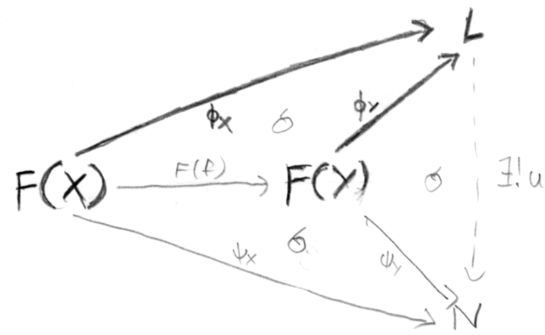L∈Ob C, together with a family φX∈MorC(F(X),L) for X∈Ob J, is a co-cone of the covariant functor F:JC  :⇔
∀f∈MorJ(X,Y) φX∘F(f)=φY
A colimit of the covariant functor F:JC is a universal co-cone (L,φX), i.e.
∀(N,(ψX)X∈Ob J) co-cone of F ∃!u∈MorC(L,N) ∀X∈Ob J u∘φX = ψX
Write: L = colim F = lim F
F:JC has a colimit ⇔ ∀N∈Ob C the covariant functor X↦MorC(F(X),N) in Jop has a limit.
⇒ MorC(colim F,N) = lim MorC(F(·),N)
"Colimits are for glueing together mathematical objects." Colimits are sometimes also known as direct limit or inductive limit. Examples of colimits include coproducts, inital objects, coequalizers, cokernels, pushouts.

Limits and colimits are uniquely determined up to a unique isomorphism, because they are inital (resp. terminal) objects in the category of cones of F.